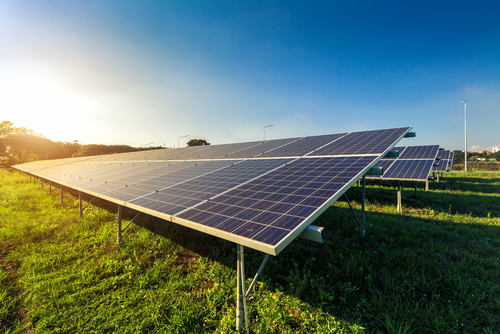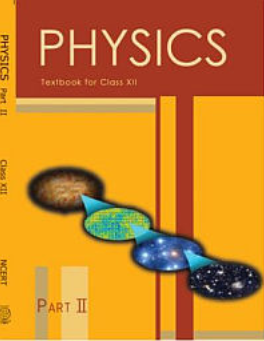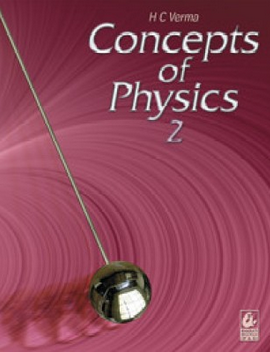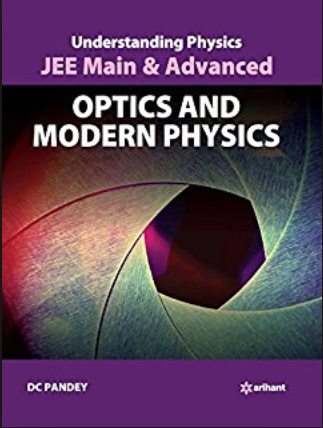Dual Nature of Matter and Radiation   Share

What is Dual Nature of Matter and Radiation

As the name suggests Dual Nature of Matter and Radiation chapter deals with the duality in the nature of matter, namely particle nature and a wave nature. Various experiments by various scientists were done to prove it. For example, light behaves both as a wave and as a particle. If you are observing phenomenon like the interference, diffraction or reflection, you will find that light behaves as a wave. However, if you are looking at phenomena like the photoelectric effect, you will find that light behaves as a particle. You must have heard about solar energy in your earlier classes and how it can be a good alternative to the non-renewable sources of energy. We can use solar energy as a source of electricity using solar panels. The solar panel has solar cells (semiconductors like Si and Ge) and when photons hit the solar cells the electrons get excited and due to their movement electric current is produced. So here we got to know that light constitutes of photons or in other words light contains particles which are a packet of energy.But light does not only shows particle nature it also shows the wave nature and you will get to know about it by various experiments. I hope now you understand why this chapter’s name is Dual Nature of Matter and Radiation.

Dual Nature of Matter and Radiation is one of the most important chapters from modern physics while preparing for all competitive exam because it helps you to understand the dual nature of matter. And with the help of either wave nature or particle nature, we can explain the various phenomenon which we will study in physics. This is easy to understand and a high scoring topic. The Concept of Dual Nature of Matter and Radiation and other chapters of physics are mixed in miscellaneous questions which are asked in various competitive exams.

Prepare Online for JEE Main/NEET

Crack JEE 2021 with JEE/NEET Online Preparation Program

Notes on the Dual Nature of Matter and Radiation

So we will discuss step by step about important topics from this chapter followed by an overview of this chapter. Then we will understand important formulas from this chapter. Remembering these formulas will increase your speed while question-solving.

Dual Nature of Matter and Radiation Topics

•  photoelectric effect,

• Hertz and Lenard's observations,

• Einstein’s photoelectric equation,

• Matter-wave: the wave nature of particles,

• De Broglie relation, Davisson and Germer experiment.

Dual Nature of Matter and Radiation Overview

• In this chapter, we will study the dual nature of matter which will be explained by Wave-particle duality postulates. According to this postulates, all particles exhibit both wave properties and particle properties.
• Then we will study the particle nature of the light wave. For this, we will consider light as particle or light consist of photons.
• Then we will study various characteristics of photons, like its mass, energy, momentum, etc. There are various scientific experiments which will explain the particle nature of light and Photoelectric Effect experiment is one of them.
• In photoelectric Effect experiment, the emission of electrons (photoelectron) takes place when light strikes a surface. We will understand all the procedure and results of this experiment. We will understand various terminology regarding this experiment and how they depend on each other. And based on their dependence on each other we can get different results if we change the input quantity of experiments. Einstein's Photoelectric Equation gives the relation between input and output quantity of experiments. This experiment also follows the Conservation of energy.
• After studying the particle nature of matter we will learn about the wave nature of matter. So we will learn about De - Broglie wave which is nothing but a wave associated with the moving material particles. To understand the wave nature of matter it is very important to study about its associated De - Broglie wavelength. So we will study various characteristics of De - Broglie wavelength. We will study how  De - Broglie wavelength of matter depends on various factors like its mass, frequency of the wave, etc. With the help of formula, we can also calculate values of De - Broglie wavelength for particles like electron, Deuteron, Alpha particle, etc.
• There are various scientific experiments like Davisson Germer Experiment, Frank Hertz Experiment, etc which will explain the wave nature of matter.

Dual Nature of Matter and Radiation Formulas

1. The energy of a photon-

1. Kinetic mass of the photon=
2. The momentum of photon=
3.  work function-
4. Einstein's Photoelectric Equation=

5.  De - Broglie wavelength-

How to prepare Dual Nature of Matter and Radiation

• First, you should have good command over the concepts of the Dual Nature of Matter and Radiation and you should also know how to apply them well at the time of the entrance exam or while solving questions.
• Please try to understand each concept from this chapter, with the help of theory, questions with solutions and video lectures on each important concept. For each concept practice enough problems so that you have a thorough understanding of the concept.
• Solve all the questions at home with proper concentration and try to do all calculation by yourself without seeing the solution first.
• While solving questions of Photoelectric Effect first find out the relation between work function and the energy of the incident wave and then solve for the result.
• Please have a thorough knowledge of Effect of intensity, potential, frequency on photoelectric current.
• Remember all the formulas of calculating De - Broglie wavelength. This will help you while solving questions.

Dual Nature of Matter and Radiation Tips

• Make a proper plan to prepare for this chapter and Stick to it.• Study the concept first and then start solving questions. Don't go through question directly without knowing the concept.
• Formulas from this chapter are very important from the point of view of competitive exam. So please remember them and solve lots of questions based on these formulas.
• Solve previous year question of various exam from this chapter.
• Use smart methods to solve questions.

Dual Nature of Matter and Radiation Books

For Dual Nature of Matter and Radiation, chapter concepts in NCERT are enough but you will have to practice lots of questions including previous year questions and you can follow other standard books available for competitive exam preparation like Concepts of Physics (H. C. Verma) and Understanding Physics by D. C. Pandey (Arihant Publications).

Physics Chapter wise Notes For Engineering and Medical Exams

 Chapters No. Chapters Name Chapter 1 Physics and Measurement Chapter 2 Kinematics Chapter 3 Laws of motion Chapter 4 Work Energy and Power Chapter 5 Rotational Motion Chapter 6 Gravitation Chapter 7 Properties of Solids and Liquids Chapter 8 Kinetic theory of Gases Chapter 9 Thermodynamics Chapter 10 Oscillations and Waves Chapter 11 Electrostatics Chapter 12 Current Electricity Chapter 13 Magnetic Effects of Current and Magnetism Chapter 14 Electromagnetic Induction and Alternating currents Chapter 15 Electromagnetic Waves Chapter 16 Optics Chapter 18 Atoms And Nuclei Chapter 19 Electronic devices Chapter 20 Communication Systems Chapter 21 Experimental skills

Topics from Dual Nature of Matter and Radiation

• Dual nature of radiation. ( JEE Main, COMEDK UGET, KEAM ) (1 concepts)
• particle nature of light ( JEE Main, COMEDK UGET, KEAM ) (9 concepts)
• Photoelectric effect, Hertz, and Lenard's observations ( JEE Main, COMEDK UGET, KEAM ) (11 concepts)
• Einstein's photoelectric equation ( JEE Main, COMEDK UGET, KEAM ) (4 concepts)
• Matter waves-wave nature of the particle, de Broglie relation & Davisson-Germer experiment. ( JEE Main, COMEDK UGET, KEAM ) (16 concepts)
• Particle Nature of Light ( JEE Main, COMEDK UGET, KEAM ) (2 concepts)
• Wave nature of matter ( JEE Main, COMEDK UGET, KEAM ) (3 concepts)
• Photoelectric effect ( JEE Main, COMEDK UGET, KEAM ) (3 concepts)
• Photon counts,Flux and Intensity of radiation ( JEE Main, COMEDK UGET, KEAM ) (4 concepts)

Important Books for Dual Nature of Matter and Radiation

•••Exams
Articles
Questions